Circular Motion NAT Level - 1

# Circular Motion NAT Level - 1

Test Description

## 10 Questions MCQ Test Topic wise Tests for IIT JAM Physics | Circular Motion NAT Level - 1

Circular Motion NAT Level - 1 for Physics 2023 is part of Topic wise Tests for IIT JAM Physics preparation. The Circular Motion NAT Level - 1 questions and answers have been prepared according to the Physics exam syllabus.The Circular Motion NAT Level - 1 MCQs are made for Physics 2023 Exam. Find important definitions, questions, notes, meanings, examples, exercises, MCQs and online tests for Circular Motion NAT Level - 1 below.
Solutions of Circular Motion NAT Level - 1 questions in English are available as part of our Topic wise Tests for IIT JAM Physics for Physics & Circular Motion NAT Level - 1 solutions in Hindi for Topic wise Tests for IIT JAM Physics course. Download more important topics, notes, lectures and mock test series for Physics Exam by signing up for free. Attempt Circular Motion NAT Level - 1 | 10 questions in 30 minutes | Mock test for Physics preparation | Free important questions MCQ to study Topic wise Tests for IIT JAM Physics for Physics Exam | Download free PDF with solutions
 1 Crore+ students have signed up on EduRev. Have you?
*Answer can only contain numeric values
Circular Motion NAT Level - 1 - Question 1

### A particle moves along a circle of radius (20/π)m with tangential acceleration of constant magnitude. If the speed of the particle is 80 m/s at the end of the second revolution after motion has begun. What will be the tangential acceleration in ms–2?

Detailed Solution for Circular Motion NAT Level - 1 - Question 1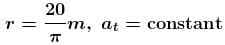n = 2 revolution
v = 80 m/s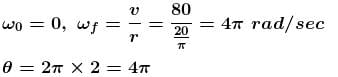from 3rd equation of motion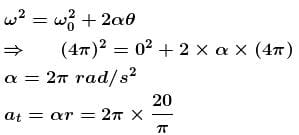= 40 m/s2

*Answer can only contain numeric values
Circular Motion NAT Level - 1 - Question 2

### In the motorcycle stunt called ‘the well of death’ the track is a vertical cylindrical surface of 18 m radius. Take the motorcycle to be a point mass and µ = 0.8. The minimum angular speed (rad/sec) of the motorcycle to prevent him from sliding down should be :

Detailed Solution for Circular Motion NAT Level - 1 - Question 2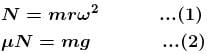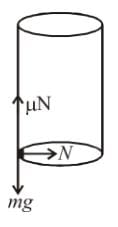From (1) and (2)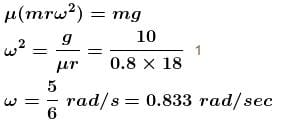*Answer can only contain numeric values
Circular Motion NAT Level - 1 - Question 3

### In the figure shown a lift goes downwards with a constant retardation. An observer in the lift observes a conical pendulum in the lift, revolving in a horizontal circle with time period 2 seconds. The distance between the centre of the circle and the point of suspension is 2.0 m. The retardation of the lift in m/s2 is :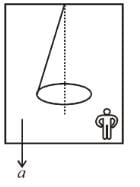Detailed Solution for Circular Motion NAT Level - 1 - Question 3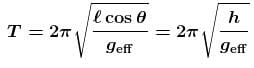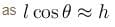geff = g + a
Put T = 2
⇒ a = 10 m/s2

*Answer can only contain numeric values
Circular Motion NAT Level - 1 - Question 4

A circular curve of a highway is designed for traffic moving at 72 km/h. If the radius of the curved path is 100 m, the correct angle (in degree) of banking of the road should be given by :

Detailed Solution for Circular Motion NAT Level - 1 - Question 4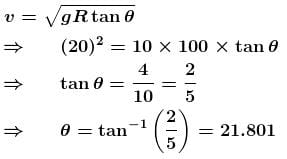*Answer can only contain numeric values
Circular Motion NAT Level - 1 - Question 5

For a body in circular motion with a constant angular velocity, the magnitude of the average acceleration over a period of a half a revolution is how many times the magnitude of its instantaneous acceleration?

Detailed Solution for Circular Motion NAT Level - 1 - Question 5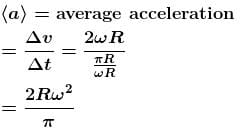Instantaneous acceleration = ω2R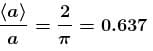*Answer can only contain numeric values
Circular Motion NAT Level - 1 - Question 6

A spot light S rotates in a horizontal plane with a constant angular velocity of 0.1 rad/s.  The spot of light P moves along the wall at a distance 3m.  What is the velocity in ms–1 of the spot  P when  θ = 45°?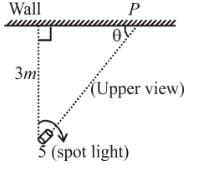Detailed Solution for Circular Motion NAT Level - 1 - Question 6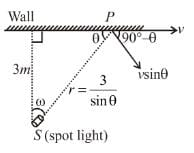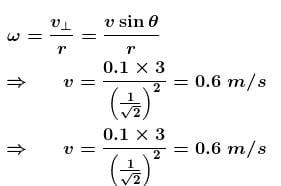*Answer can only contain numeric values
Circular Motion NAT Level - 1 - Question 7

A point P moves in counter clockwise direction on a circular path as shown in the figure. The movement of 'P'  is such that it sweeps out a length  s = t3 + 5, where  s is in metres and t  is in seconds. The radius of the path is 20 m. The acceleration in  ms–2 of 'P' when  t = 2s is nearly.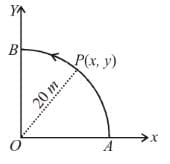Detailed Solution for Circular Motion NAT Level - 1 - Question 7

S = t3 + 5
Linear speed of the particle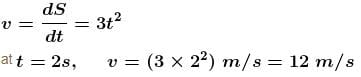Linear acceleration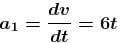at  t = 2s,     a1 = 12 m/s2

The centripetal acceleration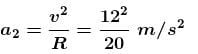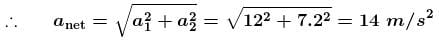*Answer can only contain numeric values
Circular Motion NAT Level - 1 - Question 8

A stone is projected from level ground at  t = 0 sec such that its horizontal and vertical components of initial velocity are 10 m/s and 20 m/s respectively. Then the instant of time (in seconds) at which tangential and normal components are acceleration of stone are same is : (neglected air resistance) g = 10m/s2

Detailed Solution for Circular Motion NAT Level - 1 - Question 8

Tangential acceleration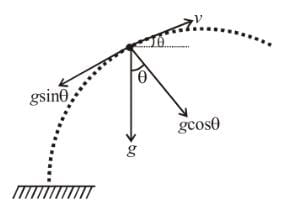Normal acceleration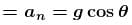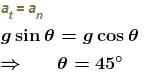During upward motion

⇒  vy = vz
uy – gt = ux
20 – 10t = 10
t = 1 sec
During downward motion
at = an
vy – vx
20 – 10t = – 10
⇒ t = 3 sec

*Answer can only contain numeric values
Circular Motion NAT Level - 1 - Question 9

A ball suspended by a thread swings in a vertical plane so that its acceleration magnitudes in the extreme and lowest positions are equal. The thread deflection angle (in degree) in the extreme position is?

Detailed Solution for Circular Motion NAT Level - 1 - Question 9

Acceleration at lowest position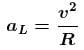From energy conservation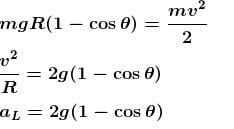acceleration at highest position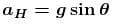according to problem aL = aH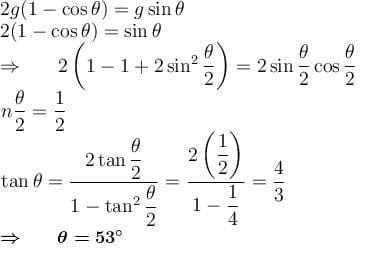*Answer can only contain numeric values
Circular Motion NAT Level - 1 - Question 10

A bird is flying in air. To take a  turn  in the  horizontal  plane of radius R = 10m with  the velocity v = 10m/s at what angle (in degree) it should bend with the horizontal.

Detailed Solution for Circular Motion NAT Level - 1 - Question 10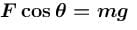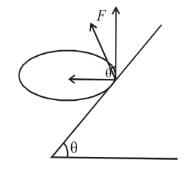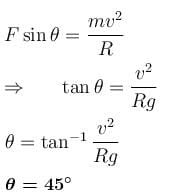## Topic wise Tests for IIT JAM Physics

217 tests
 Use Code STAYHOME200 and get INR 200 additional OFF Use Coupon Code
Information about Circular Motion NAT Level - 1 Page
In this test you can find the Exam questions for Circular Motion NAT Level - 1 solved & explained in the simplest way possible. Besides giving Questions and answers for Circular Motion NAT Level - 1, EduRev gives you an ample number of Online tests for practice

217 tests